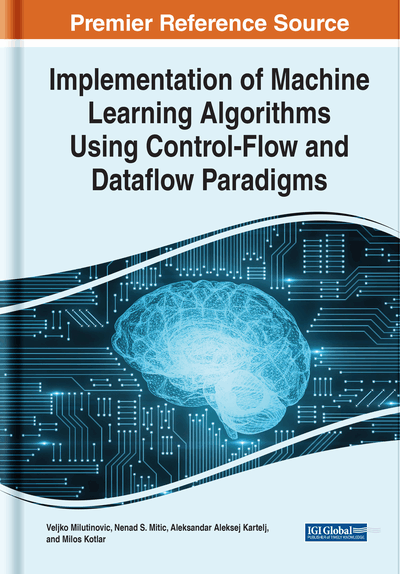# Implementation Details of Neural Networks Using Dataflow

DOI: 10.4018/978-1-7998-8350-0.ch006
Available
\$29.50
No Current Special Offers

## Abstract

This chapter presents dataflow paradigm in general and loop unrolling and data pipelines as key points for acceleration and discusses implementation details of multilayer perceptron neural networks. The iterative nature of the algorithm makes it suitable for dataflow implementation using matrix multiplication as a basic operation. Also, it presents major differences in code execution between conventional controlflow paradigm and dataflow paradigm. It is shown how part of an algorithm (feed-forward phase) can be migrated to the accelerator while the rest remains the same.
Chapter Preview
Top

In the controlflow paradigm, source code is transformed into a list of low level instructions and then loaded into memory, where the processor executes instructions and communicates with the memory, as shown in Figure 1. Memory access is slow operation and in order to optimize it there is a memory hierarchy that contains several levels of caching, where the closest level to the processor has the shortest access time.

In the dataflow paradigm, the data is retrieved from memory into the execution graph that consists of connected nodes called units, as shown in Figure 2. Each unit represents simple arithmetic or logic operation. Graph of such connected units is called an execution graph where data is streamed from the input of the graph to the output.

In the dataflow paradigm, execution goes to the lower code level where acceleration depends on the level of data reusability inside the moved loops, as well as on the number of loops and loop iterations. Dataflow accelerator used in this book is based on MaxJ programming language which is a superset of Java programming language, extended with classes for describing concepts of execution graphs. Dataflow accelerators rely on Intel FPGA cards where several accelerators are integrated and connected together. Files written in MaxJ compile first in execution graphs which contain pipelines of arithmetic and logic units. Then using third party tools provided by FPGA vendors, the compiler converts an execution graph into a file.

## Key Terms in this Chapter

Kernel: Dataflow program file that describes execution graph.

Loop Unrolling: Dataflow technique for implementing controlflow loops.

Pipeline Utilization: Optimization technique for dataflow paradigm.

FPGA: Field programmable gate array.

Manager: Dataflow program file describes data orchestration between kernel and the host machine.

## Complete Chapter List

Search this Book:
Reset